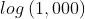## Base Log

Solve the shown logarithm:Hint
The logarithm of $$x$$ to the Base $$b$$ is defined by
$$log_b(x)=c$$$where $$b^c=x$$ . Hint 2 Special definitions when $$b=e$$ or $$b=10$$ are: • ln $$x$$ → Base = $$e$$ • log $$x$$ → Base = 10 The logarithm of $$x$$ to the Base $$b$$ is defined by $$log_b(x)=c$$$
where $$b^c=x$$ . Special definitions when $$b=e$$ or $$b=10$$ are:
• ln $$x$$ → Base = $$e$$
• log $$x$$ → Base = 10

The problem is asking to solve for $$c$$ :
$$10^c=1,000$$$Factor 10 from 1,000: $$\frac{1,000}{10}=100\rightarrow \frac{100}{10}=10$$$
$$10$$ even appears three times in $$1,000$$ . Thus,
$$log_{10}(10^3)=3$$\$
3